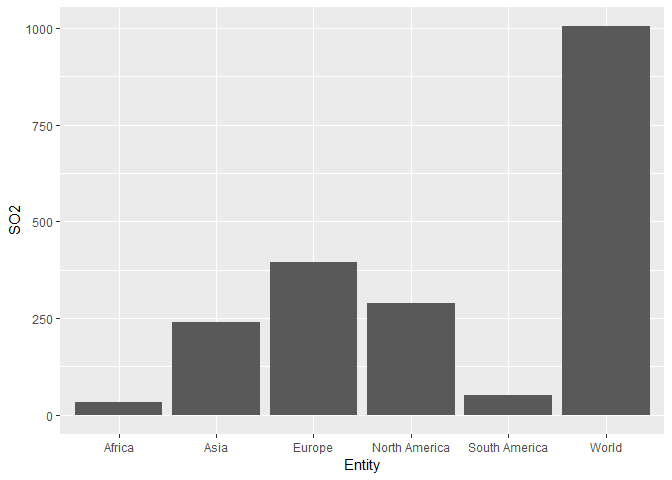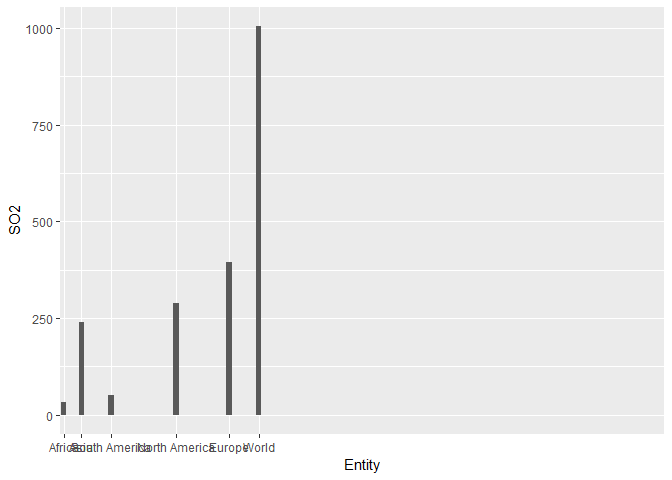# stat ="identity" and scale_x_discrete not working

``````library(ggplot2)
#set working directory
setwd("C:/Users/MOHANKRISHNA/Desktop/datascience")

data1 <- read.table(file="so.csv", sep = ",", header = TRUE, colClasses = c("factor","factor","numeric","numeric"),
na.string = "NA")

ggplot(data = data1, aes(x=Entity, y=SO2)) + geom_bar(stat = "identity")
````````````ordered <- data1[order(data1\$SO2),]

ggplot(data = data1, aes(x=Entity, y=SO2)) + geom_bar(stat = "identity") +
scale_x_discrete(limits=ordered\$Entity)
``````Where did I go wrong? Why is the stat="identity" not working by showing the same values that are in the dataset? Why is scale_x_discrete not working by arranging the bars in increasing heights?

Here is the dataset
https://ourworldindata.org/air-pollution (Click the data button under the first chart you see. Thanks.)

I renamed the million tonnes as SO2.

Are you looking for something like this?

``````dataset <- read.csv(file = 'so-emissions-by-world-region-in-million-tonnes.csv')

library(ggplot2)

ggplot(data = dataset) +
geom_col(mapping = aes(x = reorder(x = Entity,
X = SO2),
y = SO2)) +
labs(x = 'Entity')
``````Created on 2019-06-23 by the reprex package (v0.3.0)

2 Likes

Yes brother. Could you please point out the mistakes I did? Thank you so much:) Also, why were the SO2 values increasing in size when we used stat = "identity"?

I don't use `ggplot2`, hence my explanation is likely to be wrong. I'll request you to verify it, and maybe you or someone else can rectify my mistakes.

My idea is that `stat = 'identity'` has nothing to do with the order. I used `geom_col`, and you used `geom_bar(stat = 'identity')`, and these two are equivalent.

What's wrong with your code is that the `x` values are plotted in the increasing order, which is by default the alphabetical order. You want to change that using `SO2`, and hence you'll have to reorder them using that. I think `reorder` changes the ordering of the levels of the `x` argument according to the corresponding values of the `X` argument. Here, thus `Entity` is reordered according to mean value of `SO2` and their updated ordering is with respect to the `SO2` values, not with respect to the alphabetic order. Hence, plotting with these reordered `Entity` leads to the result you desire.

1 Like

I know stat = 'identity' has nothing to do with the order but it needs to mimic the values of dataset. The highest value in the data set is around 150 but if we look at the graph it's magnifying the value and showing the max value as 1000. Hope that makes sense:) Thanks for the explanation bro:)

The values being plotted are the totals. See below:

``````> with(data = dataset, expr = by(data = SO2, INDICES = Entity, FUN = sum))
Entity: Africa
 31.233
------------------------------------------------------------
Entity: Asia
 240.278
------------------------------------------------------------
Entity: Europe
 393.518
------------------------------------------------------------
Entity: North America
 289.332
------------------------------------------------------------
Entity: South America
 50.287
------------------------------------------------------------
Entity: World
 1004.648
``````
1 Like

Yup it makes sense now. Thanks again bro:)

This topic was automatically closed 7 days after the last reply. New replies are no longer allowed.